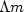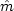Next: Ensemble Up: Resolution and random signals Previous: Bandlimited noise

# TIME-STATISTICAL RESOLUTIONIf we flipped a fair coin 1000 times, it is unlikely that we would get exactly 500 heads and 500 tails. More likely the number of heads would lie somewhere between 400 and 600. Or would it lie in another range? The theoretical value, called the mean" or the expectation," is 500. The value from our experiment in actually flipping a fair coin is called the sample mean.'' How much differenceshould we expect between the sample meanand the true mean m? Both the coin flips x and our sample meanare random variables. Our 1000-flip experiment could be repeated many times and would typically give a differenteach time. This concept will be formalized in section 11.3.5. as the variance of the sample mean,'' which is the expected squared difference between the true mean and the mean of our sample.

The problem of estimating the statistical parameters of a time series, such as its mean, also appears in seismic processing. Effectively, we deal with seismic traces of finite duration, extracted from infinite sequences whose parameters can only be estimated from the finite set of values available in these seismic traces. Since the knowledge of these parameters, such as signal-to-noise ratio, can play an important role during the processing, it can be useful not only to estimate them, but also to have an idea of the error made in this estimation.Next: Ensemble Up: Resolution and random signals Previous: Bandlimited noise
Stanford Exploration Project
10/21/1998